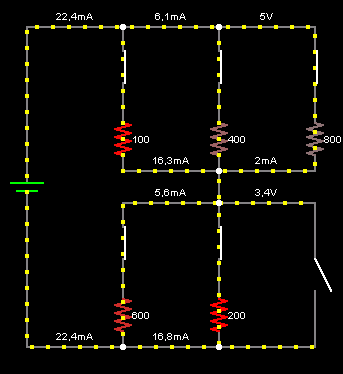# Electronic basics – ResistorsThe green color indicates positive voltage, and the gray color indicates ground (or earth). The yellow dots indicates current (in the conventional direction). The left side of the circuit shows a voltage source providing 5 volts, and the current flows through a number of switches and resistors to the right. The amount of resistance in ohms is shown to the right of each resistor.
If there is only one switch closed on top and one closed on the bottom, then there is a single path through the circuit, and by Ohm’s Law, the current will be equal to 5V divided by the total resistance through the current path.
If there are multiple current paths, you may have resistors in parallel.If all the resistors are active in the circuit and the path without resistor is open then the current flow through entire circuit is very low.To compute current you can use Ohm’s law
Electric current = Voltage / Resistance

Categorii
Articolul anterior

## Conventional Electric Current

Articolul următor